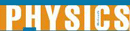Encyclopedia Article

# Ohm's Law - Research Article from World of Physics

This encyclopedia article consists of approximately 2 pages of information about Ohm's Law.
 This section contains 325 words (approx. 2 pages at 300 words per page)

Ohm's law is a relationship between the voltage across an electric circuit, the electrical resistance in the circuit, and the current in the circuit. This law is named after its discoverer, Georg Simon Ohm. Ohm found that for most electric circuits, the voltage across the circuit was equal to the current flowing through the circuit times the electrical resistance of the circuit. For the same voltage, a circuit with a low resistance will have a higher current than a circuit with a higher resistance. The voltage, properly called the potential difference, is measured in volts, and the current in amperes (amps). The resistance is therefore in volts per ampere, which is defined as ohms.

It is important to understand that Ohm's law is not a fundamental law that always applies, such as the law of gravity. Rather it is an empirical law that has been found by experiment to work fairly well most of the time. There are times, however, usually in extreme cases, when Ohm's law breaks down. For example, if an extremely high voltage is applied across a circuit, Ohm's law will not predict the correct value for the current. Even though Ohm's law does not always apply it works for most everyday situations and is therefore very useful.

For example, why will a short circuit blow a fuse or circuit breaker? When a short circuit occurs, most of the electrical resistance in the circuit is bypassed. In effect, a new circuit with a very low resistance is created. So, according to Ohm's law if the resistance is very low the current must be very high. Fuses and circuit breakers are designed to protect the circuit by blowing when the current becomes too high. Hence, the short circuit will produce a current high enough to blow the fuse. As another application, electronic devices often have resistors placed in the circuit to increase the resistance and therefore limit the current.

 This section contains 325 words (approx. 2 pages at 300 words per page)
More summaries and resources for teaching or studying Ohm's Law.
CopyrightsOhm's Law from Gale. ©2005-2006 Thomson Gale, a part of the Thomson Corporation. All rights reserved.